# 目标检测之R-CNN系列

Object Detection，在给定的图像中，找到目标图像的位置，并标注出来。 或者是，图像中有那些目标，目标的位置在那。这个目标，是限定在数据集中包含的目标种类，比如数据集中有两种目标:狗，猫。 就在图像找出来猫，狗的位置，并标注出来 是狗还是猫。

1. 目标识别，识别出来目标是猫还是狗，Image Classification解决了图像的识别问题。
2. 定位，找出来猫狗的位置。

## R-CNN

2012年AlexNet在ImageNet举办的ILSVRC中大放异彩，R-CNN作者受此启发，尝试将AlexNet在图像分类上的能力迁移到PASCAL VOC的目标检测上。这就要解决两个问题：

• 如何利用卷积网络去目标定位
• 如何在小规模的数据集上训练出较好的网络模型。

R-CNN目标检测的思路：

• 给定一张图片，从图片中选出2000个独立的候选区域(Region Proposal)
• 将每个候选区域输入到预训练好的AlexNet中，提取一个固定长度（4096）的特征向量
• 对每个目标（类别）训练一SVM分类器，识别该区域是否包含目标
• 训练一个回归器，修正候选区域中目标的位置：对于每个类，训练一个线性回归模型判断当前框是不是很完美。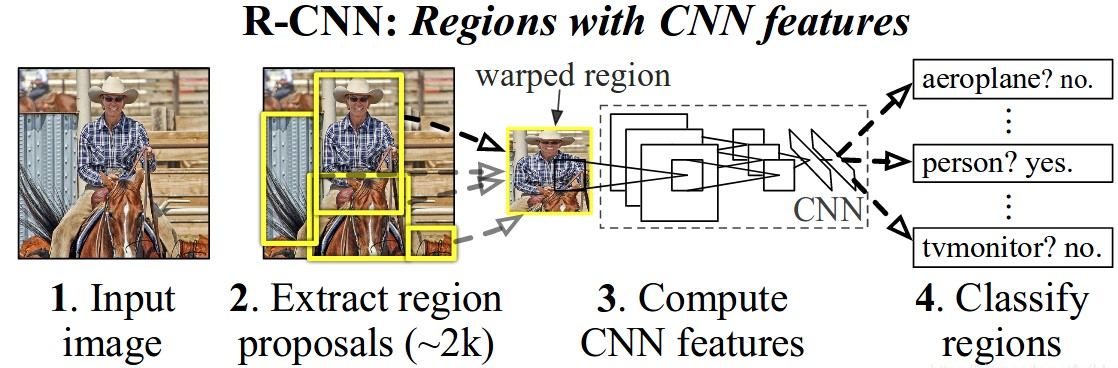### 训练

R-CNN进行目标检测的训练流程:

• 使用区域生成算法，生成2000个候选区域，这里使用的是Selective search.
• 对生成的2000个候选区域，使用预训练好的AlexNet网络进行特征提取。
• 将候选区域变换到网络需要的尺寸($227 \times 227$)。 在进行变换的时候，在每个区域的边缘添加$p$个像素，也就是手工的添加个边框，设置$p = 16$
• 改造预训练好的AlexNet网络，将其最后的全连接层去掉，并将类别设置为21（20个类别，另外一个类别代表背景）.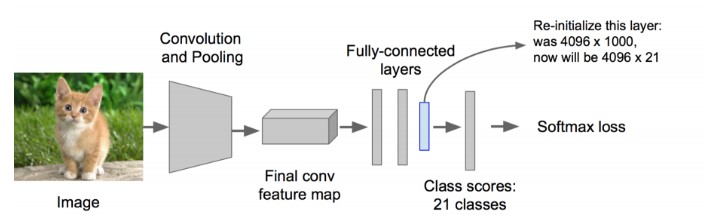这样一个候选区域输入到网络中，最终得到一个$4096 \times 21$的特征。
• 利用上面提取到的候选区域的特征，对每个类别训练一个SVM分类器（而分类）来判断，候选框里物体的类别，是给类别就是positive，不是就是negative。比如，下图针对狗的SVM分类器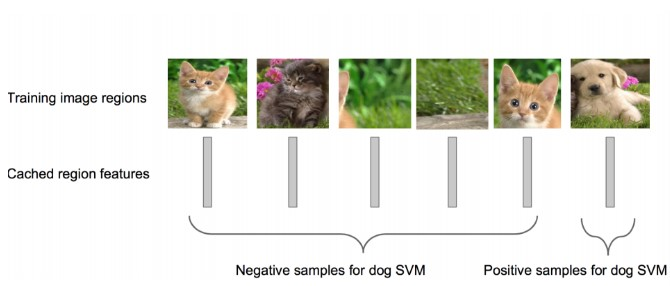狗的SVM分类器，就要能判断出某个候选区域是不是包含狗，包含狗了那就是Positive；不包含就是Negative.这里有个问题是，假如候选区域只是框出来了某个类的一部分，那要怎么来标注这个区域呢。在R-CNN中，设定一个IOU的阈值，如果该区域与Ground truth的IOU低于该阈值，就将给区域设置为Negative。阈值设置为0.3。
• 对于面只是得到了每个候选框是不是包含某个目标，其得到的区域位置不是很准确。这里需要再训练一个线性回归模型判断，候选区域框出的目标是不是完美。对于某个类别的SVM是Positive的候选区域，来判断其框的目标区域是不是很完美。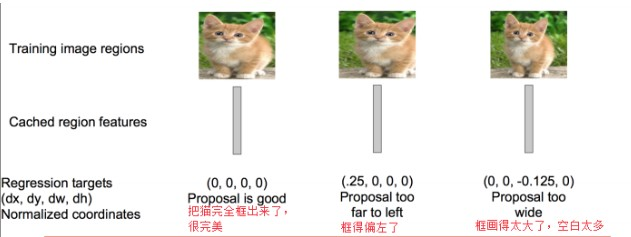### 缺点

• 训练分为多个步骤，比较繁琐。 需要微调CNN网络提取特征，训练SVM进行正负样本分类，训练边框回归器得到正确的预测位置。
• 训练耗时，中间要保持候选区域的特征，5000张的图片会生成几百G的特征文件。
• 速度慢
• SVM分类器和边框回归器的训练过程，和CNN提取特征的过程是分开的，并不能进行特征的学些更新。

## Fast R-CNN

R-CNN虽然取得了不错的成绩，但是其缺点也很明显。Ross Girshick在15年推出Fast RCNN，构思精巧，流程更为紧凑，大幅提升了目标检测的速度。同样使用最大规模的网络，Fast RCNN和RCNN相比，训练时间从84小时减少为9.5小时，测试时间从47秒减少为0.32秒。在PASCAL VOC 2007上的准确率相差不大约在66%-67%之间。

Fast RCNN主要是解决RCNN的问题的

• 测试训练速度慢，主要是提取候选区域的特征慢
R-CNN首先从测试图中提取2000个候选区域，然后将这2000个候选区域分别输入到预训练好的CNN中提取特征。由于候选区域有大量的重叠，这种提取特征的方法，就会重复的计算重叠区域的特征。在Fast-RCNN中，将整张图输入到CNN中提取特征，在邻接时在映射到每一个候选区域，这样只需要在末尾的少数层单独的处理每个候选框。
• 训练需要额外的空间保存提取到的特征信息
RCNN中需要将提取到的特征保存下来，用于为每个类训练单独的SVM分类器和边框回归器。在Fast-RCNN中，将类别判断和边框回归统一的使用CNN实现，不需要在额外的存储特征。

### Fast R-CNN的结构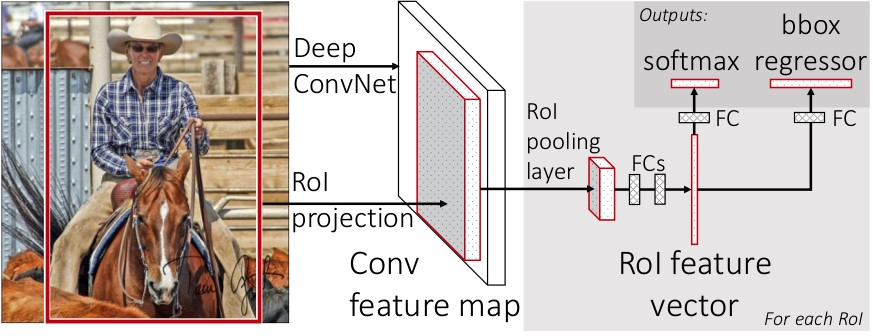Fast R-CNN网络将整个图像和一组候选框作为输入。网络首先使用几个卷积层（conv）和最大池化层来处理整个图像，以产生卷积特征图。然后，对于每个候选框，RoI池化层从特征图中提取固定长度的特征向量。每个特征向量被送入一系列全连接（fc）层中，其最终分支成两个同级输出层 ：一个输出个类别加上1个背景类别的Softmax概率估计，另一个为个类别的每一个类别输出四个实数值。每组4个值表示个类别的一个类别的检测框位置的修正。

### ROI 池化层

ROI池化层前面的网络层是对整幅图像提取特征得到多个Feature Map。ROI池化层的输入就是这多个Feature Map以及多个ROI（候选区域），这里的ROI是一个矩形框，由其左上角的坐标以及宽高组成的四元组$(r,c,h,w)$定义。

ROI池化层使用最大池化将输入的Feature Map中的任意区域（ROI对应的区域）内的特征转化为固定的$H \times W$的特征图，其中$H$$W$是超参数。 对于任意输入的$h \times w$的ROI，将其分割为$H \times W$的子网格，每个子网格的大小为$\frac{h}{H} \times \frac{w}{W}$。如下，取得$2\times 2$的特征图

1. 输入的Feature Map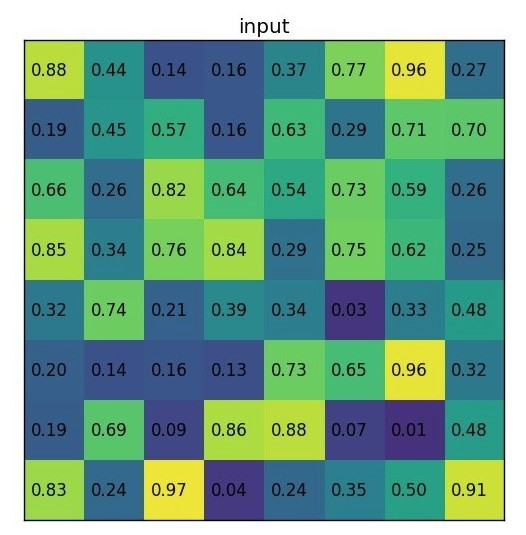2. ROI投影到Feature Map上的左上角的坐标为$(0,3)$,宽高为$(7,5)$，在Feature Map上位置如下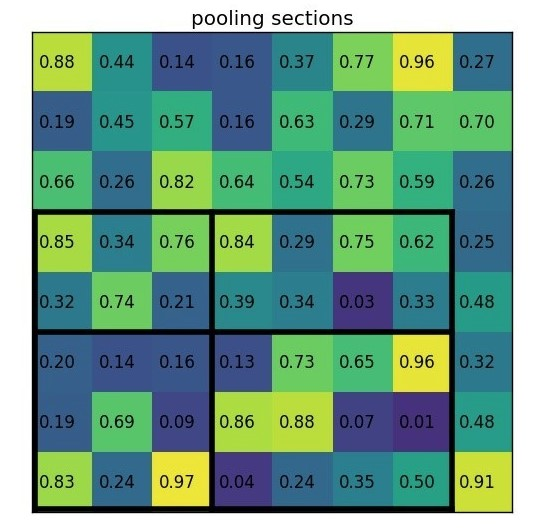3. 对每个子网格做最大池化操作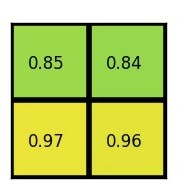ROI池化层的池化操作同标准的池化操作是一样的，每个通道都单独执行。

### 预训练网络

• 使用ROI池化层代替预训练网络的最后的池化层，并将超参$H,W$设置为和网络第一个全连接兼容的值，例如VGG16，设$H = W = 7$
• 原网络的最后一个全连接层替换为两个同级层:$K + 1$个类别的SoftMax分类层和类别的边框回归层。
• 网络的输入修改为两个：图像的列表以及相对应的ROI的列表

### 训练微调

R-CNN中的特征提取和检测部分是分开进行的，使用检测样本进行训练的时候无法更新特征提取部分的参数。SPPnet也不能更新金字塔层前面的卷积层权重，这是因为当批量训练的样本来自不同的图片时，，反向传播通过SPP层时十分低效。Fast R-CNN则可以使用反向传播的方法更新整个网络的参数。

Fast R-CNN提出一个高效的训练方法，可以在训练过程中发挥特征共享的优势。在Fast R-CNN训练过程中随机梯度下降(SGD)的mini-batch是分层采样的，首先取$N$张图像，然后从每张图片采样$\frac{R}{N}$个RoI。来自同一张图片的RoI在前向和后向传播中共享计算和内存。这样就可以减少mini-batch的计算量。例如$N=2，R=128$，这个训练模式大概比从128个不同的图像采样1个RoI（这就是R-CNN和SPPnet的训练方式）要快64倍。

Fast R-CNN有两种输出：

• 分类的Softmax输出，对于每个RoI输出一个概率，$p = {p_0,p_1,\dots,p_k}$$k + 1$个类，包括一个背景类别。
• 边框回归：$t^k = (t_x^k,t_y^k,t_w^k,t_h^k)$。 其中，$k$类别的索引，$t_x^k,t_y^k$是相对于候选区域尺度不变的平移，$t_w^k,t_h^k$相对于候选区域对数空间的位移。

$L(p,u,t^u,v) = L_{cls}(p,u) + \lambda [u \ge 1]L_{Ioc}(t^u,v)$

$L_{cls}(p,u) = -\log (p_u)$

$L_{Ioc}(t^u,v)$是边框回归的损失函数，

\begin{align*} L_{Ioc}(t^u,v) &= \sum_{i \in {x,y,w,h}}smooth_{L_1}(t_i^u - v) \end{align*}

$smooth_{L_1}(x) = \left\{ \begin{array}{cc} 0.5 x^2 & if |x| < 1 \\ |x|-0.5 & otherwise\end{array}\right.$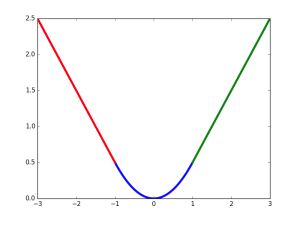### Truncated SVD for faster detection

$Y = W \cdot X$

$W \approx U \Sigma_t V^T$

### Summary

Fast R-CNN是对R-CNN的一种改进

1. 卷积不再是对每个region proposal进行，而是直接对整张图像，这样减少了很多重复计算。原来RCNN是对每个region proposal分别做卷积，因为一张图像中有2000左右的region proposal，肯定相互之间的重叠率很高，因此产生重复计算。
2. 用ROI pooling进行特征的尺寸变换，因为全连接层的输入要求尺寸大小一样，因此不能直接把region proposal作为输入
3. 将regressor放进网络一起训练，每个类别对应一个regressor，同时用softmax的全连接层代替原来的SVM分类器。

## Faster R-CNN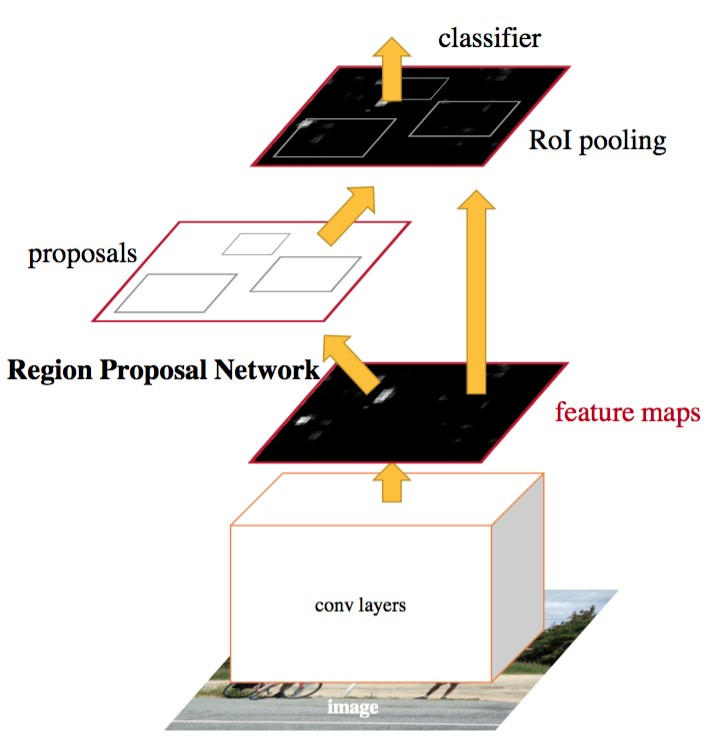Faster R-CNN的网络有4部分组成：

• Conv Layers 一组基础的CNN层，由Conv + Relu + Pooling组成，用于提取输入图像的Feature Map。通常可以选择有5个卷积层的ZF网络或者有13个卷积层的VGG16。Conv Layers提取的Feature Map用于RNP网络生成候选区域以及用于分类和边框回归的全连接层。
• RPN，区域检测网络 输入的是前面卷积层提取的Feature Map，输出为一系列的候选区域。
• RoI池化层 输入的是卷积层提取的Feature Map 和 RPN生成的候选区域RoI，其作用是将Feature Map 中每一个RoI对应的区域转为为固定大小的$H \times W$的特征图，输入到后面的分类和边框回归的全连接层。
• 分类和边框回归修正 输入的是RoI池化后RoI的$H \times W$的特征图，通过SoftMax判断每个RoI的类别，并对边框进行修正。

• 将样本图像整个输入到Conv Layers中，最后得到Feature Map。
• 将该Feature Map输入到RPN网络中，提取到一系列的候选区域
• 然后由RoI池化层提取每个候选区域的特征图
• 将候选区域的特征图输入到用于分类的Softmax层以及用于边框回归全连接层。

Faster R-CNN的4个组成部分，其中Conv Layers，RoI池化层以及分类和边框回归修正，和Fast R-CNN的区别不是很大，其重大改进就是使用RPN网络生成候选区域。

### 卷积层 Conv Layers

• 所有的卷积层都使用$3\times3$的卷积核，步长为1，并对边缘做了填充$padding=1$。这样对于输入$W \times W$的图像，通过卷积后，其输出尺寸为$(W - 3 + 2 * padding) / 1 + 1 = W$，也就是通过卷积层图像的尺寸并不会变小。
• 池化层都是用$2 \times 2$的池化单元，步长为2。对于$W \times W$的图像，通过池化层后，其输出的尺寸为$(W - 2) / 2 + 1 = W / 2$，也就是通过一个池化层图像的尺寸会变为输入前的$1 /2$。

Conv Layers的13个conv层并不会改变图像的尺寸，而有4个池化层，每个池化层将输入缩小为原来的$1/2$,则对于$W \times W$的输入，Conv Layers输出的Feature Map的宽和高为$W / 16 \times W /16$，也就是输入尺寸的$1/16$。 有了这个Feature Map相对于原始输入图像的宽高比例，就可以计算出Feature Map中的每个点对应于原图的区域。

### 区域检测网络 RPN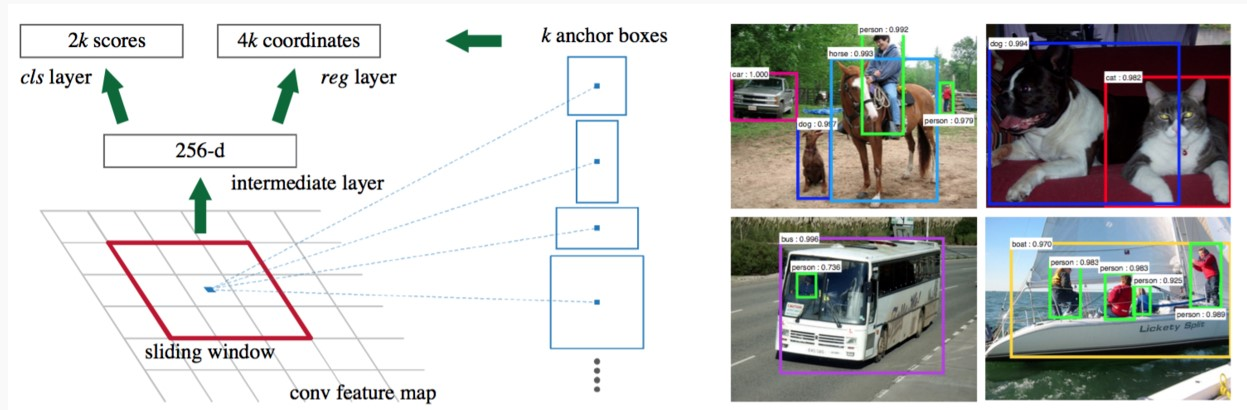RPN输入的是前面Conv Layers提取图像的Feature Map，输出有两部分：

• 候选区域的位置信息（一个4维元组）
• 候选区域对应的类别（二分类，背景还是前景）。

• 候选区域在原始输入图像的位置信息
• 每个候选区域对应的Feature Map，用于分类。

#### Anchor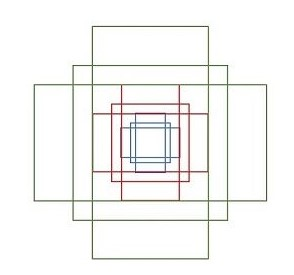$ceil(800/16) \times ceil(600 /16 ) = 50 \times 38$

• 首先将该点映射回原图的坐标为$(5 \times 16,5 \times 16) = (90,90)$
• 选择一个形状和面积的组合，例如尺度为$128 \times 128$,形状为$W:H = 1:1$
• 在原图上以$(90,90)$为中心，计算符合上述形状的区域的坐标$(x_1,y_1,x_2,y_2)=(36,36,154,154)$

\begin{align*} x_1 &= 90 - 128 / 2 = 90 - 64 = 26 \\ x_2 &= 90 + 128 / 2 = 90 + 64 = 154 \\ y_1 &= 90 - 128 / 2 = 90 - 64 = 26 \\ y_2 &= 90 + 128 / 2 = 90 + 64 = 154 \end{align*}

#### 训练

• 与任意GT区域的IoU大于0.7
• 与GT（Groud Truth）区域的IoU最大的anchor（也许不到0.7)

## summary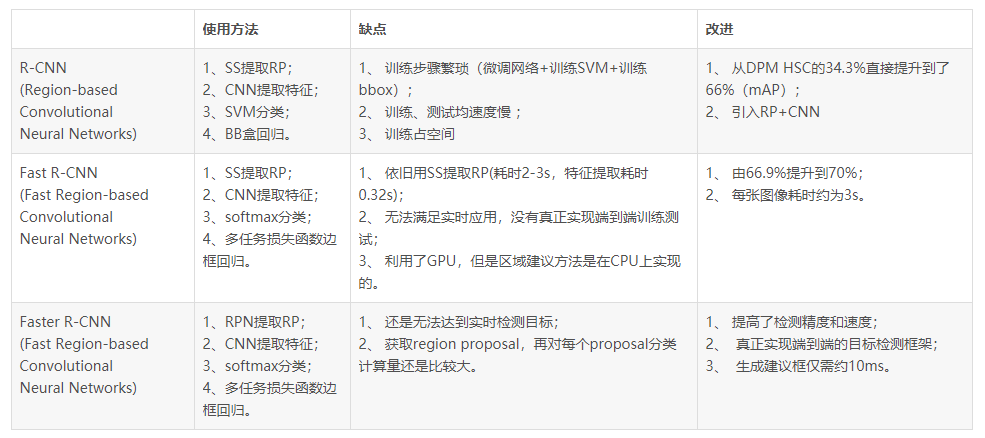posted @ 2019-02-15 15:48  Brook_icv  阅读(8533)  评论(5编辑  收藏